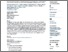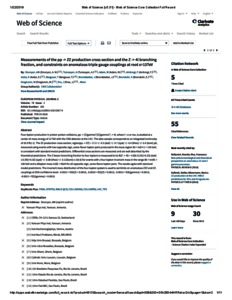Measurements of the pp -> ZZ production cross section and the Z -> 4l branching fraction, and constraints on anomalous triple gauge couplings at root s=13TeV

Sirunyan, A. M. and Tumasyan, A. R. and Adam, Wolfgang and Ambrogi, Federico and Asilar, Ece and Md. Ali, Mohd. Adli (2018) Measurements of the pp -> ZZ production cross section and the Z -> 4l branching fraction, and constraints on anomalous triple gauge couplings at root s=13TeV. The European Physical C, 78 (2). pp. 1-29. ISSN 1434-6044 E-ISSN 1434-6052PDF (The CMS project) - Published Version
Restricted to Repository staff onlyPreview
PDF (wos) - Supplemental Material

Abstract

Four-lepton production in proton-proton collisions, pp → (Z/γ ∗)(Z/γ ∗) → 4, where = e or μ, is studied at a center-of-mass energy of 13 TeV with the CMS detector at the LHC. The data sample corresponds to an integrated luminosity of 35.9 fb−1. The ZZ production cross section, σ (pp → ZZ) = 17.2 ± 0.5 (stat) ± 0.7 (syst) ± 0.4 (theo) ± 0.4 (lumi) pb, measured using events with two opposite-sign, same-flavor lepton pairs produced in the mass region 60 < m+− < 120 GeV, is consistent with standard model predictions. Differential cross sections are measured and are well described by the theoretical predictions. The Z boson branching fraction to four leptons is measured to be B(Z → 4) = 4.83+0.23 −0.22(stat) +0.32 −0.29(syst) ± 0.08(theo) ± 0.12(lumi) × 10−6 for events with a four-lepton invariant mass in the range 80 < m4 < 100 GeV and a dilepton mass m > 4 GeV for all opposite-sign, same-flavor lepton pairs. The results agree with standard model predictions. The invariant mass distribution of the four-lepton system is used to set limits on anomalous ZZZ and ZZγ couplings at 95% confidence level: − 0.0012 < f Z 4 < 0.0010, − 0.0010 < f Z 5 < 0.0013, − 0.0012 < f γ 4 < 0.0013, − 0.0012 < f γ 5 < 0.0013.

Item Type: Article (Journal) 7861/70434 pp → ZZ ; cross section; Z → 4 branching fraction; constraints on anomalous triple gauge couplings at √s = 13 TeV Q Science > Q Science (General)Q Science > QC Physics Kulliyyah of ScienceKulliyyah of Science > Department of Physics Dr Mohd Adli MD Ali 04 Feb 2019 12:03 15 Jul 2019 09:30 http://irep.iium.edu.my/id/eprint/70434View Item